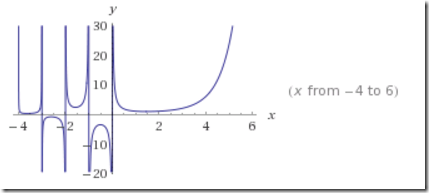## Friday, March 17, 2017

### Binomial

In a nonlinear model I needed to use the following expression in a model equation:

 $y = \binom{x}{k}p^k(1-p)^{(x-k)}$

Here $$x$$ is the only decision variable. The integer version of “n choose k” is well known:

 $\binom{n}{k} = \frac{n!}{k!(n-k)!}$

For fractional values we can generalize this to:

 $\binom{x}{y} = \frac{\Gamma(x+1)}{\Gamma(y+1)\Gamma(x-y+1)}$

where $$\Gamma(.)$$ indicates the gamma function, defined by

 $\Gamma(z) = \int_0^{\infty}x^{z-1}e^{-x} dx$In GAMS we have the binomial(x,y) function for this. The problem is that $$x$$ can become rather large, while $$k$$ is a small integer, say $$k=0,\dots,4$$. For some values of $$x$$ this leads to very inaccurate results and even overflows in subsequent calculations.

E.g.:

 scalars  x /112.8/  k /2/;parameter y;y("binomial") = binomial(x,k);y("log") = exp(loggamma(x+1)-loggamma(k+1)-loggamma(x-k+1));display y;

yields the wrong result:

 ----      8 PARAMETER y  binomial 1.0000E+299,    log         6305.520

The logarithmic version is correct.

Instead of using the logarithmic version:

 $\binom{x}{k} = \exp\left(\log\Gamma(x+1)-\log\Gamma(k+1)-\log\Gamma(x-k+1)\right)$

we can also exploit that $$k$$ is a small integer. From:

 $\Gamma(z+1)=z\Gamma(z)$

we have:

 \begin{align}\binom{x}{k}&=\frac{x(x-1)\cdots(x-k+1)\Gamma(x-k+1)}{k!\Gamma(x-k+1)}\\&=\frac{x(x-1)\cdots(x-k+1)}{k!}\\&=\frac{\displaystyle\prod_{i=1}^k (x-i+1)}{k!}\end{align}

In GAMS:

 scalars  x /112.8/  k /2/;parameter y;y("binomial") = binomial(x,k);y("log") = exp(loggamma(x+1)-loggamma(k+1)-loggamma(x-k+1));set i /1*2/;y("prod") = prod(i,x-i.val+1)/fact(k);display y;

with results:

 ----     10 PARAMETER y  binomial 1.0000E+299,    log         6305.520,    prod        6305.520

I used this last “product” version in the model.

Note that the overflow in the binomial function does not happen for all (or even most) large values:

 scalars  x /1112.8/  k /2/;parameter y;y("binomial") = binomial(x,k);y("log") = exp(loggamma(x+1)-loggamma(k+1)-loggamma(x-k+1));set i /1*2/;y("prod") = prod(i,x-i.val+1)/fact(k);display y;

gives:

 ----     10 PARAMETER y  binomial 618605.520,    log      618605.520,    prod     618605.520